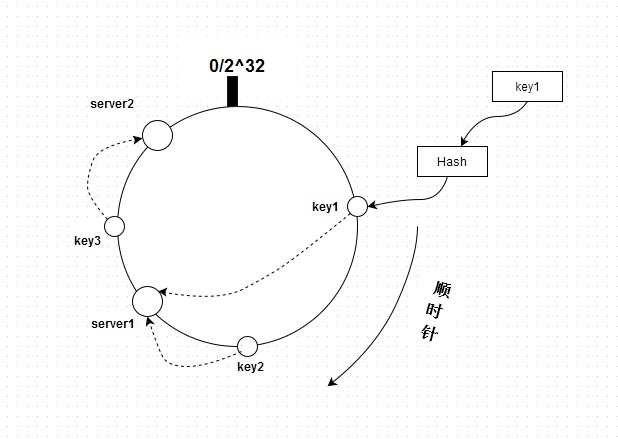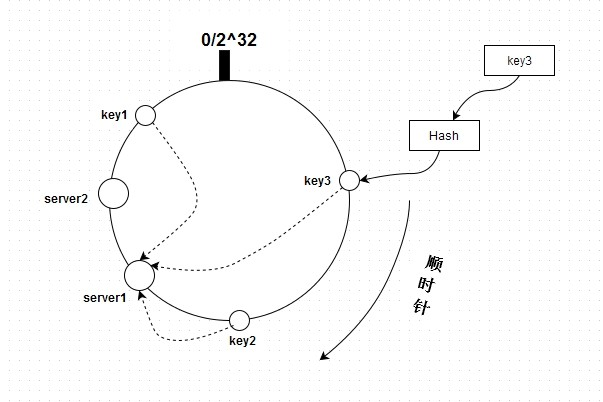# PHP: 深入了解一致性哈希

2014/02/27 12:46

## 哈希(Hash)

Hash，一般翻译做“散列”，也有直接音译为"哈希"的，就是把任意长度的输入（又叫做预映射， pre-image），通过散列算法，变换成固定长度的输出，该输出就是散列值。这种转换是一种压缩映射，也就是散列值的空间通常远小于输入的空间，不同的输入可能会散列成相同的输出，而不可能从散列值来唯一的确定输入值。

PHP内核就采用了time33算法来实现HashTable，来看下time33的定义：

hash(i) = hash(i-1) * 33 + str[i]

<?php
function myHash($str) { // hash(i) = hash(i-1) * 33 + str[i]$hash = 0;
$s = md5($str);
$seed = 5;$len  = 32;
for ($i = 0;$i < $len;$i++) {
// (hash << 5) + hash 相当于 hash * 33
//$hash = sprintf("%u",$hash * 33) + ord($s{$i});
//$hash = ($hash * 33 + ord($s{$i})) & 0x7FFFFFFF;
$hash = ($hash << $seed) +$hash + ord($s{$i});
}

return $hash & 0x7FFFFFFF; } echo myHash("却道天凉好个秋~"); $ php -f test.php
530413806

## 利用取模实现

<?php
function myHash($str) { // hash(i) = hash(i-1) * 33 + str[i]$hash = 0;
$s = md5($str);
$seed = 5;$len  = 32;
for ($i = 0;$i < $len;$i++) {
// (hash << 5) + hash 相当于 hash * 33
//$hash = sprintf("%u",$hash * 33) + ord($s{$i});
//$hash = ($hash * 33 + ord($s{$i})) & 0x7FFFFFFF;
$hash = ($hash << $seed) +$hash + ord($s{$i});
}

return $hash & 0x7FFFFFFF; } echo "key1: " . (myHash("key1") % 2) . "\n"; echo "key2: " . (myHash("key2") % 2) . "\n"; $ php -f test.php
key1: 0
key2: 0

## 一致性哈希key1、key2、key3和server1、server2通过hash都能在这个圆环上找到自己的位置，并且通过顺时针的方式来将key定位到server。按上图来说，key1和key2存储到server1，而key3存储到server2。如果新增一台server，hash后在key1和key2之间，则只会影响key1(key1将会存储在新增的server上)，其它不变。

<?php
function myHash($str) { // hash(i) = hash(i-1) * 33 + str[i]$hash = 0;
$s = md5($str);
$seed = 5;$len  = 32;
for ($i = 0;$i < $len;$i++) {
// (hash << 5) + hash 相当于 hash * 33
//$hash = sprintf("%u",$hash * 33) + ord($s{$i});
//$hash = ($hash * 33 + ord($s{$i})) & 0x7FFFFFFF;
$hash = ($hash << $seed) +$hash + ord($s{$i});
}

return $hash & 0x7FFFFFFF; } //echo myHash("却道天凉好个秋~"); echo "key1: " . myHash("key1") . "\n"; echo "key2: " . myHash("key2") . "\n"; echo "key3: " . myHash("key3") . "\n"; echo "serv1: " . myHash("server1") . "\n"; echo "serv2: " . myHash("server2") . "\n"; $ php -f test.php
key1: 351111878
key2: 1305159920
key3: 1688027782
serv1: 1003059623
serv2: 429427407key1、key2和key3都存储到了server1上，这是正确的，因为是按顺时针来定位。我们想像一下，所有的server其实就是一个排好序的数组(降序)：[server2, server1]，然后通过计算key的hash值来得到处于哪个server上。来分析下定位过程：如果只有一台server，即[server]，则直接定位，取数组的第一个元素。如果有多台server，则要先看通过key计算的hash值是否落在[server2, server1, ...]这个区间上，这个直接跟数组的第一个元素和最后一个元素比较就知道了。然后就可以通过查找来定位了。

## 利用一致性哈希实现

<?php
function myHash($str) { // hash(i) = hash(i-1) * 33 + str[i]$hash = 0;
$s = md5($str);
$seed = 5;$len  = 32;
for ($i = 0;$i < $len;$i++) {
// (hash << 5) + hash 相当于 hash * 33
//$hash = sprintf("%u",$hash * 33) + ord($s{$i});
//$hash = ($hash * 33 + ord($s{$i})) & 0x7FFFFFFF;
$hash = ($hash << $seed) +$hash + ord($s{$i});
}

return $hash & 0x7FFFFFFF; } class ConsistentHash { // server列表 private$_server_list = array();
// 延迟排序，因为可能会执行多次addServer
private $_layze_sorted = FALSE; public function addServer($server) {
$hash = myHash($server);
$this->_layze_sorted = FALSE; if (!isset($this->_server_list[$hash])) {$this->_server_list[$hash] =$server;
}

return $this; } public function find($key) {
// 排序
if (!$this->_layze_sorted) { asort($this->_server_list);
$this->_layze_sorted = TRUE; }$hash = myHash($key);$len  = sizeof($this->_server_list); if ($len == 0) {
return FALSE;
}

$keys = array_keys($this->_server_list);
$values = array_values($this->_server_list);

// 如果不在区间内，则返回最后一个server
if ($hash <=$keys || $hash >=$keys[$len - 1]) { return$values[$len - 1]; } foreach ($keys as $key=>$pos) {
$next_pos = NULL; if (isset($keys[$key + 1])) {$next_pos = $keys[$key + 1];
}

if (is_null($next_pos)) { return$values[$key]; } // 区间判断 if ($hash >= $pos &&$hash <= $next_pos) { return$values[$key]; } } } }$consisHash = new ConsistentHash();
$consisHash->addServer("serv1")->addServer("serv2")->addServer("server3"); echo "key1 at " .$consisHash->find("key1") . ".\n";
echo "key2 at " . $consisHash->find("key2") . ".\n"; echo "key3 at " .$consisHash->find("key3") . ".\n";

$php -f test.php key1 at server3. key2 at server3. key3 at serv2. 即使新增或下线服务器，也不会影响全部，只要根据hash顺时针定位就可以了。 ## 结束语 经常有人问在有多台redis server时，新增或删除节点如何通知其它节点。之所以会这么问，是因为不了解redis的部署方式。这些都是依赖一致性哈希来实现分布式的，这种实现都是由各种语言的driver去完成。所以了解一致性哈希算法的原理以及应用场合是很有必要的。 展开阅读全文 打赏 5 39 收藏 分享 ### 作者的其它热门文章 加载中你这套算法到了后面好像不对吧 首先排序要根据键名排 然后 最后返回应该是next_pos 而不应该是当前key 个人见解 2017/12/01 15:20 回复 举报 谢谢分享 2017/05/17 10:56 回复 举报 博主你好，阅读了文章，收益，谢谢！ 有个疑问：$this->_server_list 这个变量，我猜博主是想得到一个按照hash值顺序排序的数组吧，而asort($this->_server_list);得到的是原server字符串的顺序排序数组，应该是ksort($this->_server_list)吧？
2016/07/12 00:31

2016/07/10 18:54

#### 引用来自“miyae”的评论

2016/06/24 23:25

2016/06/19 16:42

2016/04/21 13:32

2015/08/27 19:39

15 评论
39 收藏
5